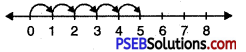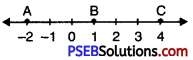# PSEB 6th Class Maths MCQ Chapter 4 Integers

Punjab State Board PSEB 6th Class Maths Book Solutions Chapter 4 Integers MCQ Questions with Answers.

## PSEB 6th Class Maths Chapter 4 Integers MCQ Questions

Multiple Choice Questions

Question 1.
How many integers are between -3 to 3?
(a) 5
(b) 6
(c) 4
(d) 3.
(a) 5Question 2.
Which of the following integer is greater than -3?
(a) -5
(b) -4
(c) 0
(d) -10.
(c) 0

Question 3.
Which of the following integers are in ascending order?
(a) -5, -9, -7, -8
(b) -9, -8, -7, -5
(c) -5, -7, -8, -8, -9
(d) -8, -5, -9, -7.
(b) -9, -8, -7, -5

Question 4.
Which of the following integers are in descending order?
(a) 3, 0, -2, -5
(b) -5, -2, 0, 3
(c) -5, 3,-2, 0
(d) -2, 0, -5, 3.
(a) 3, 0, -2, -5

Question 5.
The given number line represents:(a) 5 + 1
(b) 1 + 5
(c) 1 + 1 + 1 + 1 + 1
(d) 5 + 5 + 5 + 5 + 5.
(c) 1 + 1 + 1 + 1 + 1Question 6.
3 less than -2 =
(a) -5
(b) -6
(c) 5
(d) 6.
(a) -5

Question 7.
(-2) + 8 =
(a) -6
(b) -10
(c) 10
(d) 6.
(d) 6.

Question 8.
Which of the following statements is true about the given number line:(a) Value of A is greater than value of B.
(b) Value of A is greater than value of C.
(c) Value of B is less than value of C.
(d) Value of C is less than value of B.
(c) Value of B is less than value of C.

Question 9.
(-7) + (-12) + 11 =
(a) -19
(b) 30
(c) -23
(d) -8.
(d) -8.Question 10.
15 – (-12) + (-27) =
(a) 0
(b) -54
(c) -24
(d) 54.
(a) 0

Question 11.
What is the number of integers between -4 and -1?
(a) 3
(b) 4
(c) 5
(d) 6.
(b) 4

Question 12.
What is the number of integers between -8 and -2?
(a) 3
(b) 4
(c) 5
(d) 6.
(c) 5

Question 13.
Which is the largest integers among -7, -6, -5, -4 and -3?
(a) -6
(b) -5
(c) -4
(d) -3.
(d) -3.Question 14.
Which is the smallest integer among -3, -2, 0 and 1?
(a) -3
(b) -2
(c) 0
(d) 1.
(a) -3

Question 15.
The value of (-7) + (-9) + 4 + 16 is:
(a) 36
(b) 22
(c) 4
(d) 27.
(c) 4

Fill in the blanks:

Question (i)
The sum of (-9) + (+4) + (-6) + (+3) is …………. .
– 8

Question (ii)
The successor of -5 is ……………. .
– 4

Question (iii)
-19 + …………. = 0.
19Question (iv)
100 + …………. = 0.
– 100

Question (v)
50 + (-50) = ……………. .
0

Write True/False:

Question (i)
-8 is to the right of -10 on number line. (True/False)
True

Question (ii)
-100 is to the right of -50 on number line. (True/False)
False

Question (iii)
Smallest negative integer is -1. (True/False)